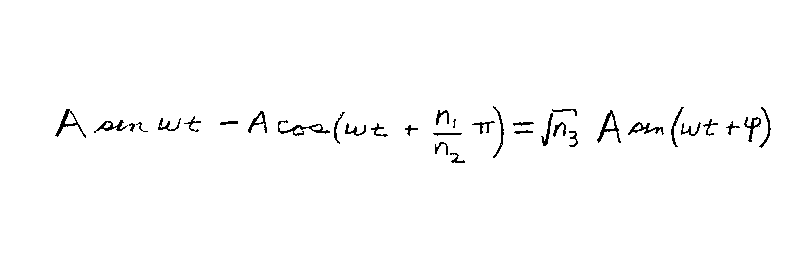Problem C18: One source produces a wave that varies in time as y1=A sin(ωt) at a certain position. Another source produces a wave that varies in time as y2=-A cos(ωt + (n1/n2)π) at the same position. What is the net amplitude at this position due to the two sources if they are coherent? If the displacement is: y(t)=y1+y2= √ n3 A sin(ωt + φ), what is n3? Note that n1, n2 and n3 are unitless.n1 = n2 = Input n3:

If you are currently in my class, you can record your grade by entering your name and student ID number (without the leading zeros) below and clicking on "record grade".
 First Name = Last Name = ID = Problem: